# Minus Numbers

## Alice Visits the Snow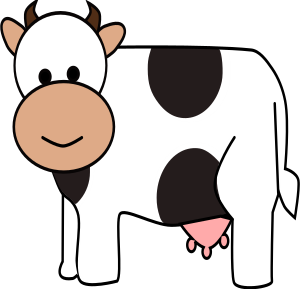Alice the cow has decided that she'd like to learn to ski. So, she heads off to Perisher Valley with her friend, Annabelle. When she gets there, she realises that she's never been so cold in her life. There's all this white stuff around and it's freezing cold to touch. What's going on?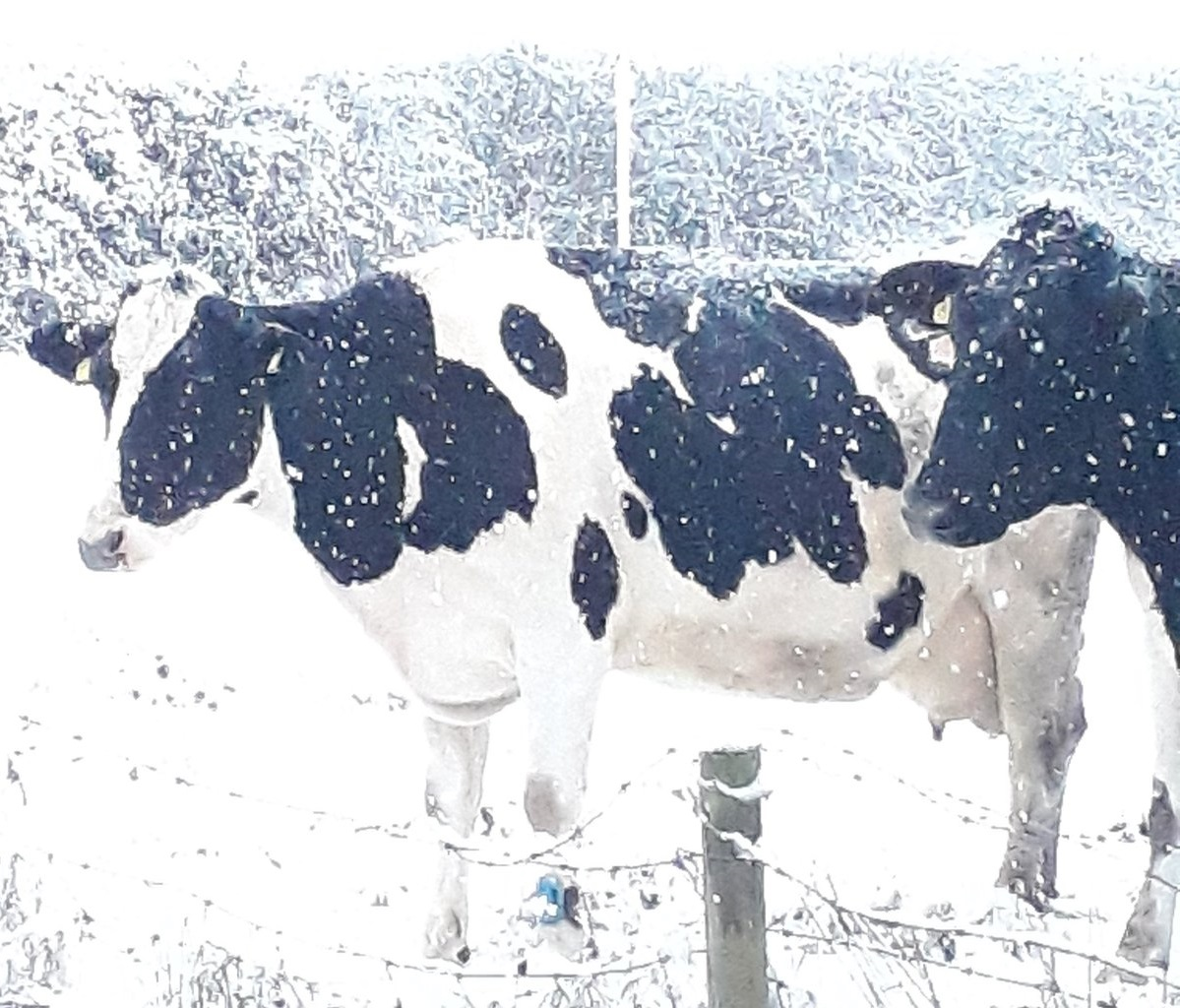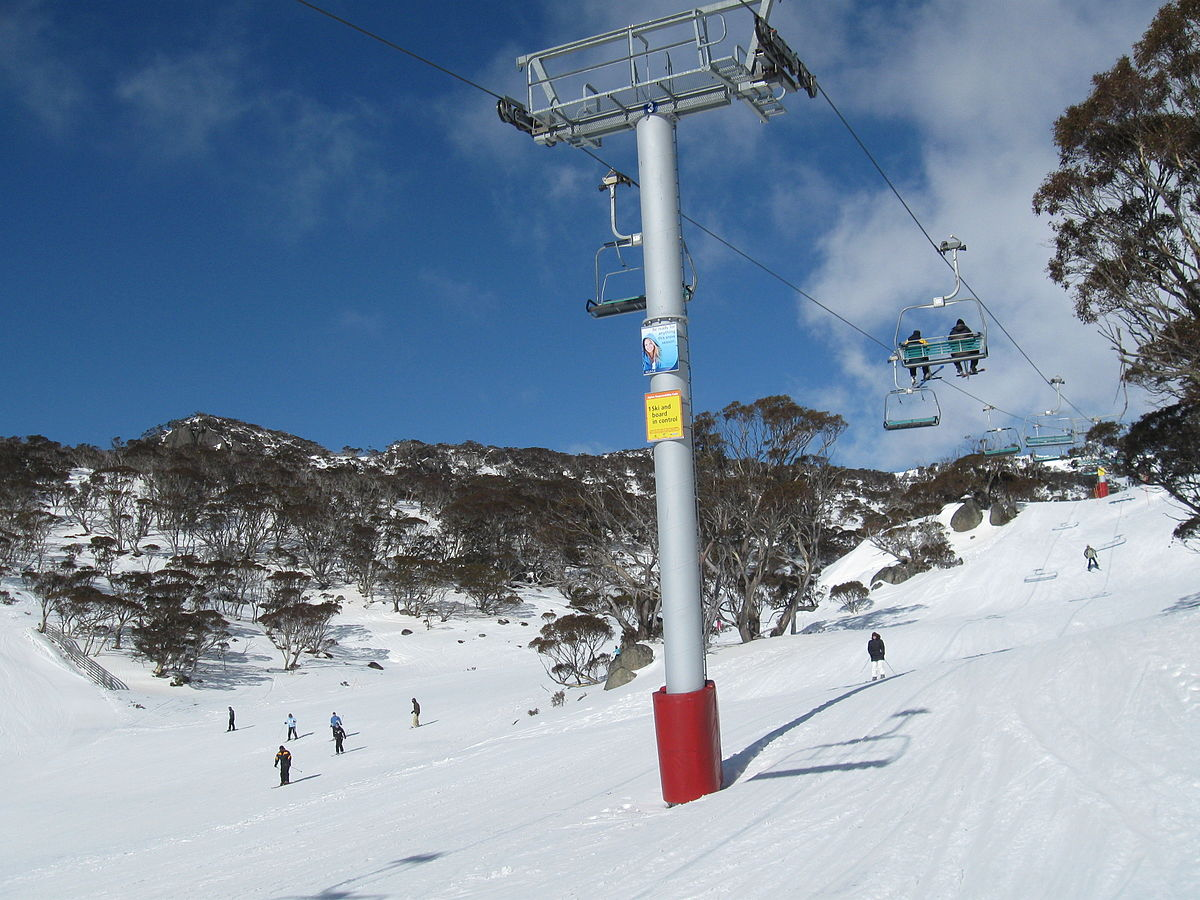Well, Alice, that white stuff is called snow. We need snow to ski on. It's made out of frozen water, and for water to freeze, it has to be cold.

Water freezes at $0^\circ$ Celsius. For snow to stick around, the temperature has to be less than $0^\circ$ Celsius. Temperatures less than $0^\circ$ Celsius are very cold indeed.

Have a look at the thermometer. Do you notice how the alcohol (the red stuff) is actually below the zero mark? It looks like it's halfway between the zero and the mark that says $-15$, so the temperature must be about $-8^\circ$ Celsius. That's pretty cold!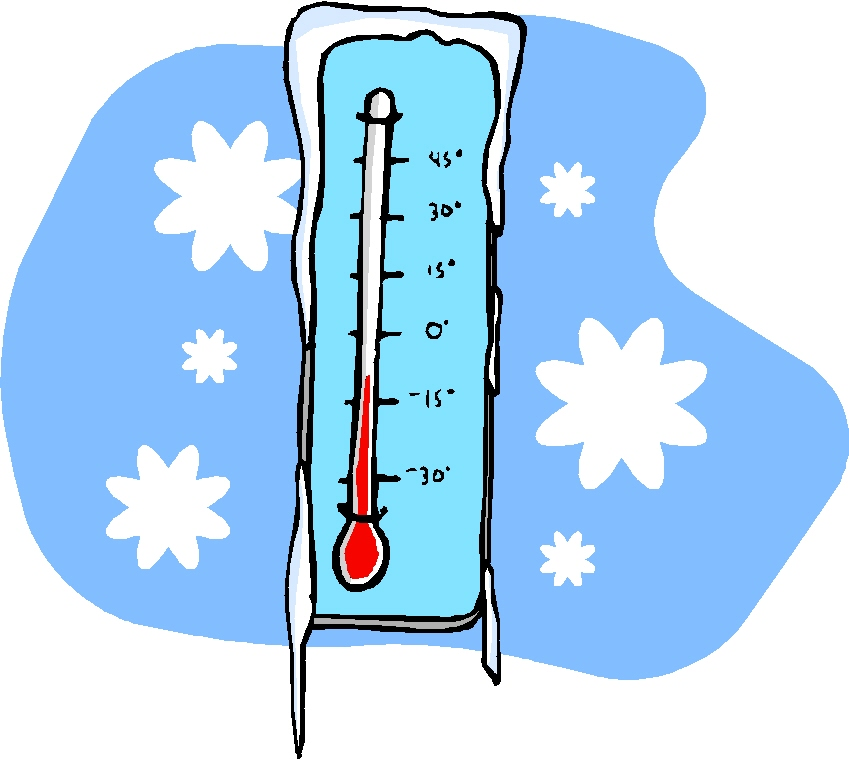Did you notice the "minus" in front of the $8$ in that temperature? It's there because we're measuring a temperature below zero. If you look at the thermometer in the picture, you can actually see minus signs in front of the $15$ and the $30$. $-8$, $-15$ and $-30$ are all examples of minus numbers. Let's find out more about them.

## The Numbers Less than Zero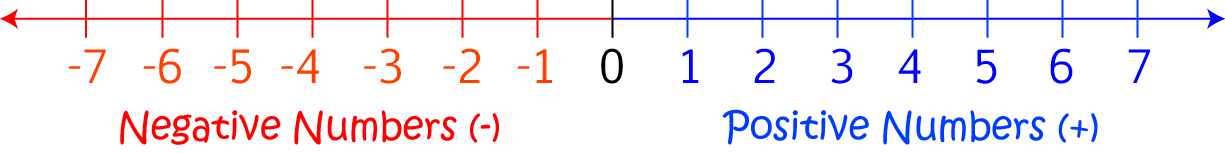The number line above looks a little bit different from the number lines that we've seen until now. It goes on to the left of zero, and it is labelled with numbers like $-1, -2, -3$ and so on. The left part of the number line looks like a reflection of the right part, except that the numbers all have minus signs in front of them. The numbers on the left hand part of the number line are the minus numbers. Another name for them is the negative numbers.

The minus numbers are the numbers that are less than zero. They might be whole numbers, fractions, or decimals. Some examples are $-8$, $-15$, and $-20$. Other examples are $-12 \dfrac{1}{2}$ and $- 2.7$.

## Uses for Minus Numbers

We've just seen that they're useful for measuring very cold temperatures like the ones that Alice is experiencing at Perisher Valley. They tell us that the temperature is below the temperature at which water freezes, so it must be very cold indeed. Negative numbers have other uses as well.

Have a think about when you do subtraction with trading. You might say something like "$3 - 5$ can't be done, so we trade $10$ with the tens column and find $13 -5 = 8$". Sometimes there isn't a tens column to trade with, but we still need to give an answer. The answer will be a minus number.

For example, suppose you need to give your friend $7$ lollies, but you only have $5$. The number of lollies you have left will be $5 - 7 = -2$, a minus number. This minus number means that you owe your friend $2$ lollies. Next time you have lollies, don't forget to give your friend the $2$ you owe them! Banks use minus numbers to tell people that they owe them money.

### Conclusion

Don't worry too much about the minus numbers at this stage. For now, it's enough to know that they exist, and that they are useful. Alice needs to know that the minus numbers exist, and that they're less than zero so that she can tell how cold it is at Perisher Valley.

Eventually, you'll learn to compare, add, subtract, multiply and divide minus numbers. Arithmetic with minus numbers is much the same as normal arithmetic, but there are some new rules to follow. It's a good idea to get more used to working with the numbers that aren't minus numbers first. We call the numbers that we've been working with until now the positive numbers.

### Description

This mini book covers the core of Math for Foundation, Grade 1 and Grade 2 mathematics including

1. Numbers
3. Subtraction
4. Division
5. Algebra
6. Geometry
7. Data
8. Estimation
9. Probability/Chance
10. Measurement
11. Time
12. Money
13. and much more

This material is provided free of cost for Parent looking for some tricks for their Prekinder, Kinder, Prep, Year 1 and Year 2 children

### Learning Objectives

These lessons are for kids aged 4-8 with the core objective to expose their brains to concepts of addition, subtraction, division, algebra and much more.

Author: Subject Coach
You must be logged in as Student to ask a Question.